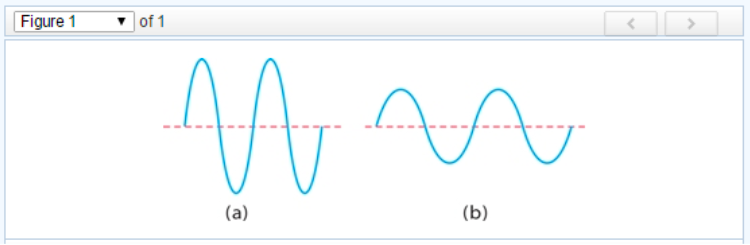# Problem: Two electromagnetic waves are represented belowPart A. Which wave has the greater intensity?a. wave (a)b. wave (b)Part B. Which wave corresponds to higher-energy radiation?a. wave (a)b. wave (b)Part C. Which wave represents yellow light, and which represents infrared radiation?a. wave (a) represents yellow lightb. wave (b) represents yellow light

###### FREE Expert Solution

Part A

The wave with greater intensity is the one with greater amplitude.

Wave (a) has a greater amplitude than wave (b).

99% (292 ratings)###### Problem Details

Two electromagnetic waves are represented belowPart A. Which wave has the greater intensity?
a. wave (a)
b. wave (b)

Part B. Which wave corresponds to higher-energy radiation?
a. wave (a)
b. wave (b)

Part C. Which wave represents yellow light, and which represents infrared radiation?
a. wave (a) represents yellow light
b. wave (b) represents yellow light